### Showing videos fromEddie Woo with a total of 4,120 videos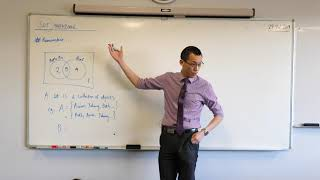10:21
Set Notation (1 of 3: Intersection, union, size)
11h13m ago06:40
Using the Mean (1 of 2: Finding an extra value)
2D11h ago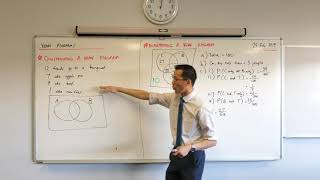07:26
Venn Diagrams for Probability (3 of 3: Constructing from information)
3D11h ago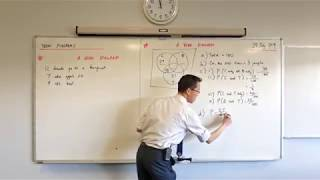10:50
Venn Diagrams for Probability (2 of 3: Interpreting a given diagram)
4D11h ago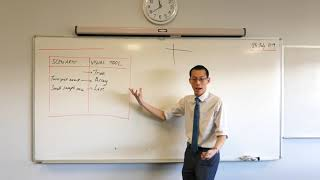08:11
Venn Diagrams for Probability (1 of 3: Comparing visual tools)
5D11h ago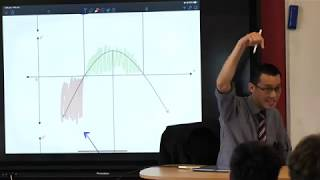07:19
Determining Derivatives from Graphs (3 of 3: Reversing the process)
6D10h ago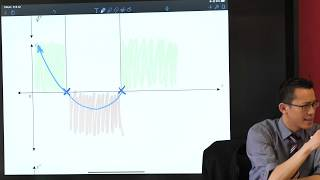07:22
Determining Derivatives from Graphs (2 of 3: Considering sign of the gradient)
7D10h ago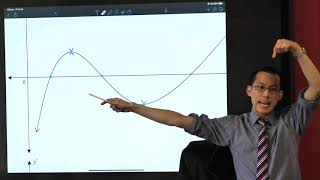07:15
Determining Derivatives from Graphs (1 of 3: Identifying major features)
8D10h ago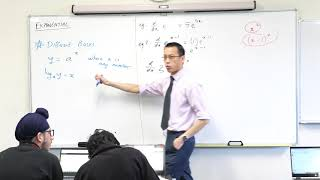06:09
Calculus of Exponential Functions (4 of 4: Differentiating with non-e bases)
10D10h ago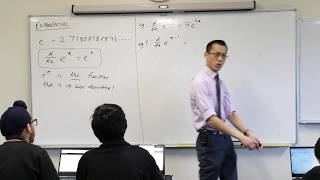06:08
Calculus of Exponential Functions (3 of 4: Basic differentiation examples)
11D10h ago09:10
Calculus of Exponential Functions (2 of 4: The importance of 2.718...)
12D10h ago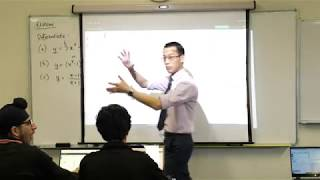09:14
Calculus of Exponential Functions (1 of 4: Considering derivatives visually)
13D10h ago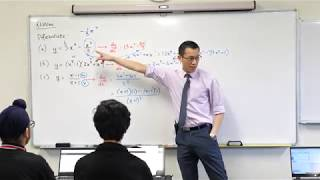09:38
Review of Basic Differentiation (2 of 2: Considering derivatives visually)
14D10h ago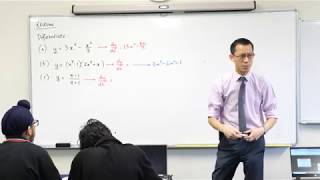06:36
Review of Basic Differentiation (1 of 2: Polynomials, Products, Quotients)
15D10h ago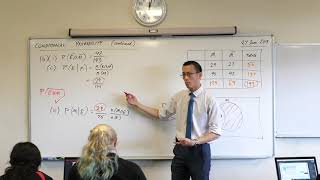08:05
Conditional Probability (7 of 7: Describing regions with set notation)
16D10h ago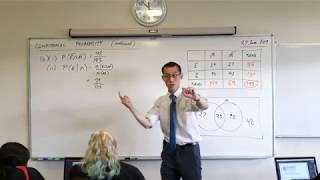09:40
Conditional Probability (6 of 7: Analysis with a Venn diagram)
17D10h ago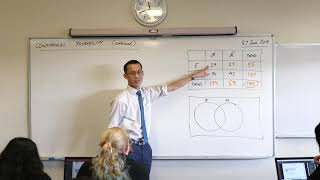07:35
Conditional Probability (5 of 7: Analysis with a table)
18D10h ago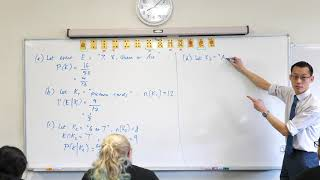08:06
Conditional Probability (4 of 7: Using formal condition notation)
19D10h ago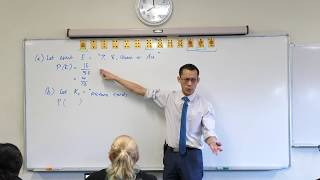06:48
Conditional Probability (3 of 7: Analysing a deck of cards)
20D12h ago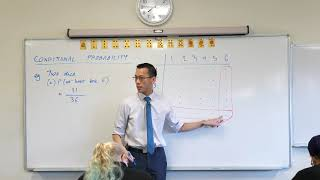07:52
Conditional Probability (2 of 7: Analysis with an array)
21D10h ago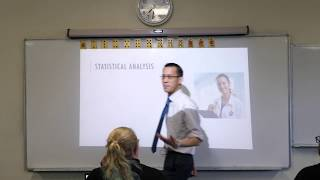10:24
Conditional Probability (1 of 7: A surprising example)
22D10h ago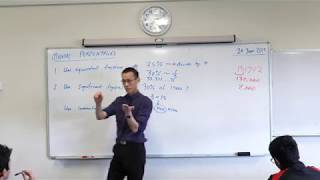10:50
Strategies for Mentally Calculating Percentages
24D10h ago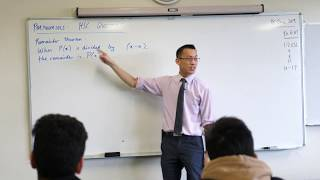07:52
HSC Polynomial Question (Determining a linear remainder)
25D10h ago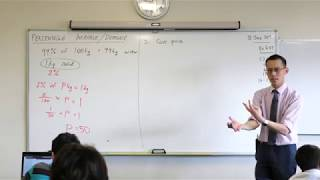04:56
Percentage Increase/Decrease (2 of 2: Example question)
26D10h ago07:35
Percentage Increase/Decrease (1 of 2: The Potato Paradox)
27D10h ago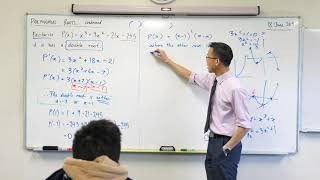05:03
Using Multiplicity of Roots (2 of 2: Completing the polynomial factorisation)
28D10h ago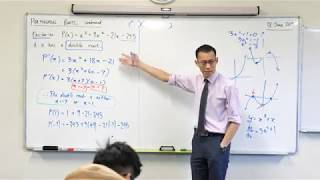11:56
Using Multiplicity of Roots (1 of 2: Locating a double root with calculus)
29D10h ago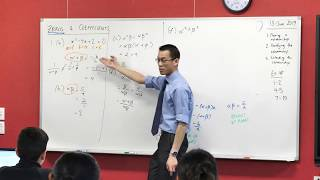07:52
Zeroes & Coefficients (5 of 5: Obtaining more complicated results)
1m1D ago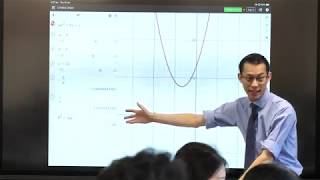09:35
Zeroes & Coefficients (3 of 5: Experimenting visually)
1m3D ago10:33
Zeroes & Coefficients (2 of 5: Generalising the result)
1m4D ago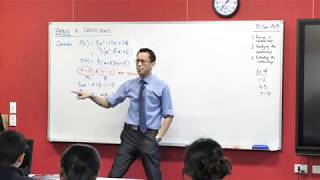07:52
Zeroes & Coefficients (1 of 5: Noticing the relationship)
1m5D ago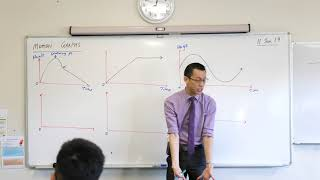07:08
Motion Graphs (1 of 2: Cannon Man's Displacement)
1m7D ago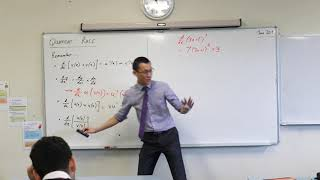13:54
Quotient Rule (1 of 2: Proof from product & chain rule)
1m10D ago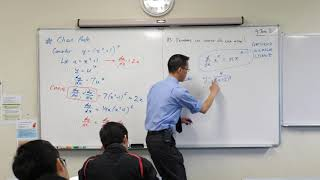12:37
Leibniz's Derivative Notation (3 of 3: Introducing the chain rule)
1m14D ago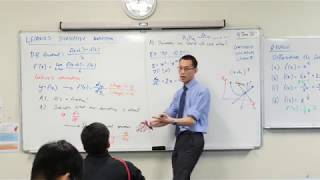09:19
Leibniz's Derivative Notation (2 of 3: Finding equation of a tangent)
1m15D ago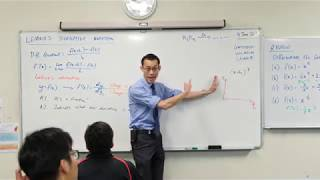13:10
Leibniz's Derivative Notation (1 of 3: Overview)
1m16D ago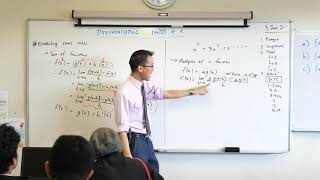08:14
Basics of Calculus, continued (2 of 2: Multiples of functions, constant function)
1m18D ago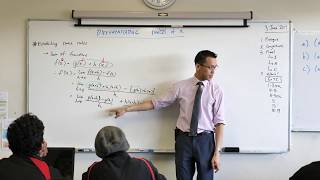07:35
Basics of Calculus, continued (1 of 2: Sum of functions)
1m19D ago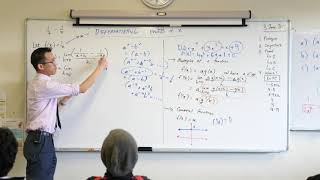08:44
Power Rule for Differentiation (4 of 4: Hyperbola)
1m20D ago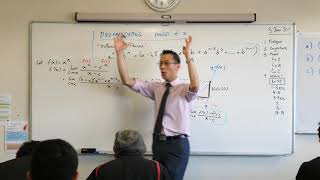10:27
Power Rule for Differentiation (3 of 4: Derivation of rule)
1m21D ago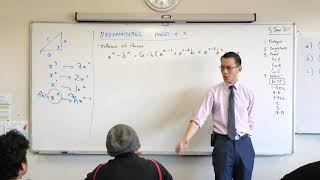12:57
Power Rule for Differentiation (2 of 4: Background knowledge)
1m22D ago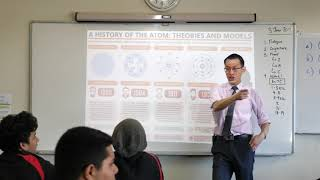08:49
Power Rule for Differentiation (1 of 4: Conjecture)
1m23D ago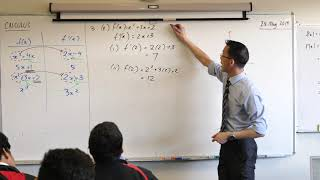07:04
Basics of Calculus (5 of 5: Locating a tangent)
1m26D ago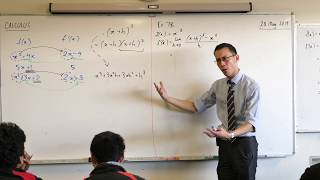10:47
Basics of Calculus (4 of 5: Considering the gradient function visually)
1m27D ago06:35
Basics of Calculus (3 of 5: Observing patterns in first principles)
1m28D ago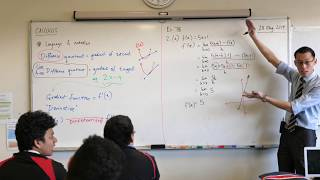05:36
Basics of Calculus (2 of 5: Example of using first principles)
1m29D ago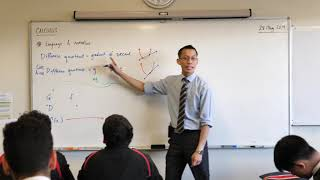07:25
Basics of Calculus (1 of 5: Foundational language & notation)
1m30D ago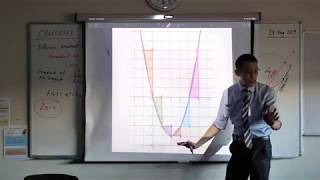11:58
Prologue to Calculus (5 of 5: Gradient of the tangent)
2m0D ago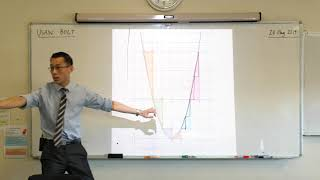09:03
Prologue to Calculus (4 of 5: Exploring a parabola)
2m1D ago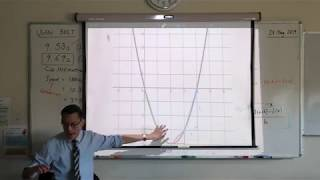08:33
Prologue to Calculus (3 of 5: The difference quotient)
2m2D ago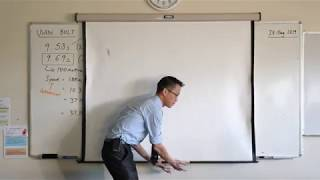09:59
Prologue to Calculus (2 of 5: How can we measure more accurately?)
2m3D ago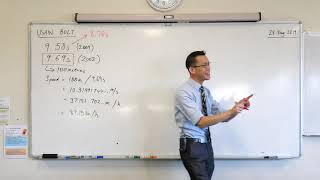10:05
Prologue to Calculus (1 of 5: How fast did Usain Bolt run?)
2m4D ago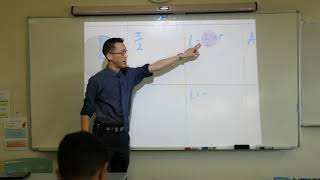11:56
Circular Measure: Arc Length, Sector & Segment Area Formulas
2m6D ago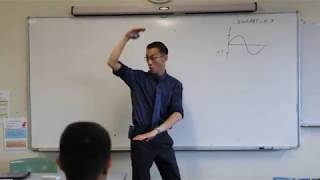09:46
3D Trig Three Observer Problem (4 of 4: Resolving the ambiguous case)
2m7D ago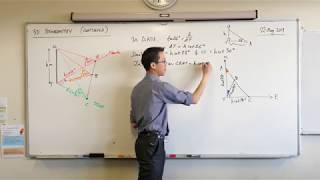10:03
3D Trig Three Observer Problem (3 of 4: Calculating ground angles)
2m8D ago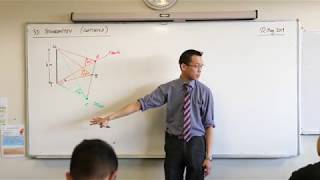08:39
3D Trig Three Observer Problem (2 of 4: Determining ground distances)
2m9D ago